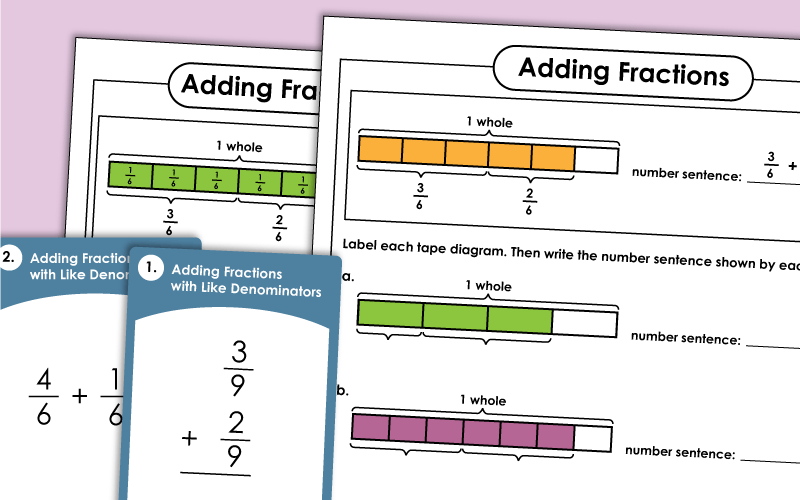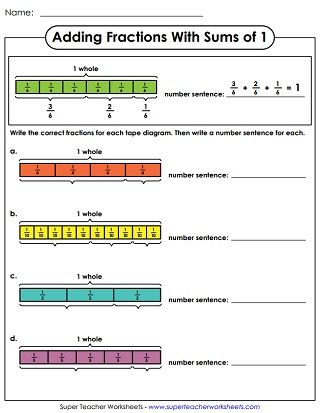# Adding Fractions & Mixed Numbers

Worksheets for practicing addition of fractions.  Includes adding fractions with the same denominator (easy) and addition with unlike denominators (harder).  There are also worksheets for adding mixed numbers.This practice sheet has 12 horizontal problems. Add fractions with like denominators and simplify the answer. (example: 2/7 + 3/7)
Add fractions with like denominators using tape diagrams. All problems written horizontally.
Create your own tape diagrams to find the sums of fraction pairs. Fractions have like denominators.
Adding fractions with the same denominators, No simplifying (All answers already in lowest terms), All vertical problems. (example:  4/6 + 1/6 = 5/6)
Worksheet for adding fractions with denominators that are the same; Requires simplifying; Vertical problems. (example:  1/6 + 2/6 = 3/6)
Worksheet for adding fractions with the same denominators, Horizontal and vertical problems. (example:  2/6 + 3/6 = 5/6)
Practice a range of fraction addition problems with this variety worksheet.
This file has 30 task cards that you can cut apart. Use them for classroom scavenger hunts, learning centers, exit slips, or peer help sessions.
This printable has basic, horizontal problems with like denominators. Includes a mix of addition and subtraction problems.
Answers greater than 1, Requires converting from improper fractions to mixed number, All horizontal problems. (example:  5/8 + 4/8 = 1 1/8)
Another worksheet for adding fractions with like denominators, All horizontal problems. (example:  3/8 + 2/8 = 5/8)
On top of the page is an array of shapes with fractions in them. Students should add pairs of fractions in similar shapes. Example: Find the sum of the the fractions in the triangles.
Write the missing numbers on each fraction number bond. (example: 1/5 + 1/5 + 1/5 = 3/5)
Students solve addition problems with fractions with the same denominators. They then use their answers to solve the riddle.

Worksheet for adding fractions with denominators that are different; Only one addend needs to be changed; Vertical problems; Requires simplifying. (example:  1/3 + 1/6 = 1/2)
Another worksheet for adding fractions with denominators that are different. (example:  1/4 + 1/2 = 3/4) Vertical problems.
This worksheet has twelve horizontal fraction addition problems. All problems have unlike denominators.
Practice adding fractions with different denominators with this variety worksheet.
This page has polygons with fractions in them. Students add similar polygons together. For example: Find the sum of the fractions in the hexagons.
Choose a fraction from each circle at the top of the page. Then find the sum.
Adding fractions with two unlike denominators, Requires simplifying, Vertical problems, Advanced level

Add the mixed numbers with like denominators; Vertical problems; Requires simplifying
Find sums and differences for the pairs of numbers. All problems written horizontally.
Use this worksheet to help students practice a range of different types of mixed-number-addition problems.

Adding mixed numbers with different denominators. There are no improper fractions in the addends or the answers.
Add pairs of mixed numbers with unlike denominators. This page has 12 problems, and there is space for students to show their work.
A set of 30 task cards for adding mixed numbers with unlike denominators.
With this worksheet, students can practice solving several different types of mixed number addition problems.
At the top of the page, students are given a set of mixed numbers with basic shapes around them. Students add numbers with similar shapes. For example: Add the numbers in the trapezoids.
Here are some horizontal problems for adding and subtracting mixed numbers with unlike denominators. All problems are horizontal.
These addition problems have mixed numbers with improper fractions in the sums. A few also have improper fractions as addends.
Subtracting Fractions

This page has worksheets on subtracting fractions and mixed numbers. Includes like and unlike denominators.

Fraction Worksheets

Worksheets for teaching basic fractions, equivalent fractions, simplifying fractions, comparing fractions, and ordering fractions. There are also worksheets on addition, subtraction, multiplication, and division of fractions.

## Worksheet ImagesMy Account
Site Information